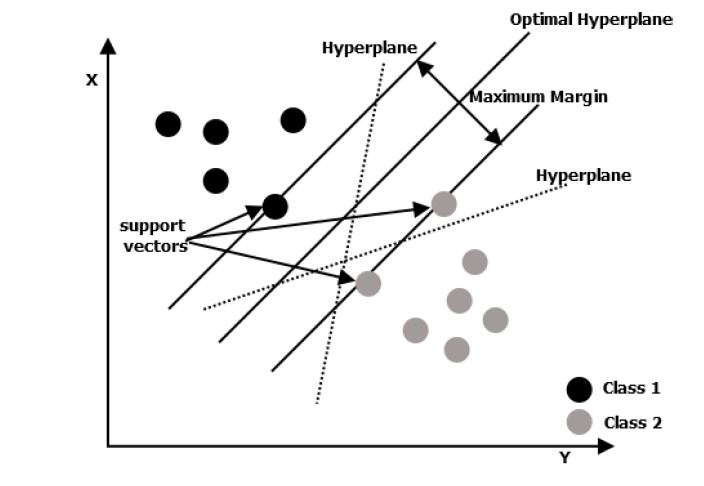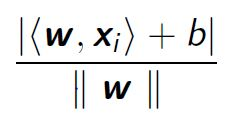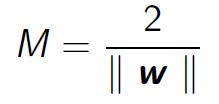# Support Vector Machine : How and What ?

May 20, 2022 by Louis Detry | 1150 views

Among the many machine learning methods available today, support vector machine method is one of the most widely used methods for classification problems. But how does it works? What are the pros and cons?

# Definition

Support vector machine were first proposed by Vapnik in 1998. The principle of this classification method is based on the notion of margin and on the search for a separating hyperplane of dimension p-1 in the feature space of dimension p between two classes in such a way that the distance (called margin) between the hyperplane and the closest data points of each class is maximized. The points located on the margin are called support vectors and the solution is a linear combination of these support vectors.This approach is based on minimizing the classification risk rather than on optimal classification.

From a mathematical point of view, find the best separating hyperplane consists of solving an optimisation problem.

The simplest problem is to separate data with 2 features (two-dimension data). the data are assumed to be linearly separable by the hyperplane :This hyperplane allows separating the space into 2 zones:And the two margins are respectively:From a mathematical point of view the distance between a point and a straight line can be expressed as:The distance to be maximized between the two margins is, therefore:This amounts to finding w and b that minimize :with the following constraints :

# Kernel function

When points are not linearly separable, SVMs use appropriate kernel functions to transpose these points into higher dimensional spaces called feature spaces.The choice of kernel functions is very important. For example, there is the radial basis function (RBF) also called Gaussian kernel which can be used to classify complex regions. Other kernels exist such as polynomial, laplacian, or hyperbolic tangent kernels.

SVMs can be divided into 2 classes based on their kernel function:

• Linear SVM
• Non-linear SVM

In linear SVMs, the training data is separated by the hyperplane using a linear kernel function. When the data is more complex and not linearly separable, the use of a non-linear separator will give poor results, which is why it is preferred, as explained above, to transpose the data into higher dimensional feature spaces in order to find a linear hyperplane there.

# Not only for binary classification

SVMs can also be used to solve multi-class classification problems. This can be done with two different strategies :

• One-versus-one
• One-versus-all

For the first strategy, a binary SVM is built for each pair of classes and the class with the most votes is selected. For the other one, the problem is divided into binary problems for each class. These are supervised learning methods.

# Conclusion

SVMs have a very good generalisation capability and are very useful when the number of features is high and the number of data points is low. However, the execution of SVM methods is computationally intensive and the algorithmic complexity is O(n*n) where n is the number of instances, which is relatively high. The performance depends on the choice of kernel functions and the parameters of these functions. The training time is also high but SVMs are methods that are resistant to noise in the data